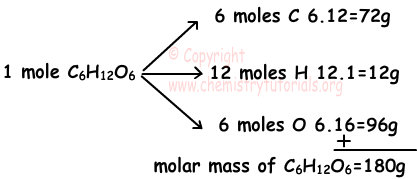## Chemical Reaction Stoichiometry with Examples

Chemical Reaction Stoichiometry with Examples

Example: If 90 g of C2H6 is burn with enough O2, find how many moles of H2O, CO2 are produced and volume of O2.(H=1, C=12, O=16)

Solution:

We first find moles of C2H6;

Molar mass of C2H6=2.12+6.1=30 g/mol

nC2H6=90/30=3 moles

When compounds including C and H atoms are burn, CO2 and H2O are produced. Now we write chemical reaction and balance it.

C2H6 + O2 → CO2 + H2O

To balance number of C atoms in both sides we add 2 in front of CO2, and to balance number of H atoms in both sides we add 3 in front of H2O.

C2H6 + O2 → 2CO2 + 3H2O

Now we must balance number of O atoms in both sides, we have 7 O atoms in right side and 2 O atoms in left side, we add 7/2 in front of O2 molecule to balance number of O atoms in both sides. Final balanced reaction becomes;

C2H6 + 7/2O2 → 2CO2 + 3H2O

Relation between amounts of matters is;

When 1 mole of C2H6 is burn 7/2 moles O2 is used and 2 moles CO2 and 3 moles H2O are produced.

When 1 mole of C2H6 is burn      3 moles H2O are produced

3 moles of C2H6 are burn                  X

____________________________________________________

X=9 moles of H2O are produced.

Molar mass of H2O=2.1+16=18g

So; 9H2O=9.18=162 g H2O are produced.

When 1 mole of C2H6 is burn      2 moles CO2 are produced

3 moles of C2H6 are burn                  X

____________________________________________________

X=6 moles of CO2 are produced.

Molar mass of CO2=12+2.16=44 g

So; 6CO2=6.44=264 g CO2 are produced.

When 1 mole of C2H6 is burn      7/2 moles O2 are used

3 moles of C2H6 are burn                  X

____________________________________________________

X=21/2 moles of O2 are used.

1 mole of O2 is             22,4 liters

21/2 moles of O2 is        X liters

__________________________________________

X=235,2 liters O2 are used for burning 3 moles C2H6

Empirical and Molecular Formula:

Molecular formula is real formula, and it shows how many moles of atoms exist in one mole of compound. For example, C6H12O6 is real formula of glucose. 1 mole glucose includes 6 moles C atoms, 12 moles H atoms and 6 moles O atoms. On the contrary, empirical formula is the simplified form of molecular formula and it shows ratio between atoms of compound. For example, empirical formula of glucose is CH2O. Empirical formula does not give us as much information as molecular formula. More over, it can belong to more than one compound, such as CH2 is empirical formula of C2H4, C3H6, C4H8. Relation between molecular formula and empirical formula is;

(Molecular Formula)=n(Empirical Formula)

Percent Composition:

Percent compositions of elements are calculated by using molecular formula of compound. Try to understand it by given example below.

Percent of element by mass=(mass of element in 1 mole compound)/(mass of one mole compound).100

Example: Find percent compositions of elements in C6H12O6. (C=12, H=1, O=16)

Percent of C by mass=(mass of C in one mole compound)/(mass of one mole compound).100C %=100X72/180

C % =40

H %=100.12/180

H % =6,7

O % =100.96/180

O % =53,3

Example: If empirical formula of organic compound is CH2O, which ones of the following statements are true;

(C= 12, H=1, O=16)

Solution:

I. Compound contains 40 % C by mass

II. Compound contains 50 % H by mole

III. If molecular mass of compound is 60 g, its molecules contain 8 atoms.

I. Mass of empirical formula=12+2.1+16=30 g

C + H + O

Percent mass of C=12/30x100=40

I is true

II. Compound contains 1 mole C, 2 mole H and 1 mole O, total number of moles=4 moles

Percent mole of H=(2moles H)/((4moles)x100=50 %

II is true

III. Molar formula =n(empirical formula)

Molar mass=n(empirical mass)

60=n30

n=2

Thus; molecular formula becomes;

C2H4O2 and it has 8 atoms.

Example: If there are 6 g X in 7,4 g X3N2 compound, find atomic mass of X.(N=14)

Solution: There are 6 g X in 7,4 g compound. Ratio of masses of elements;

mx/mN=6/1,4

This ratio is equal to ratio between elements in one mole of compound.

mx/mN=3X/2.14

6/1,4=3X/2.14

X=40 g/mole

Limiting Reactant Problems

Example: Find amount of C2H6 produced by using 0, 3 moles C2H2 and 0,4 moles H2 using following chemical reaction.

C2H2(g) + 2H2(g) → C2H6(g)

Solution:

We should firs find limiting matters to calculate amount of products.

1 mole C2H2 reacts with 2 mole H2

0,3 mole C2H2 reacts with X mole H2

————————————————————

X=0,6 moles H2 is needed

However we have 0,3 moles H2, so H2 is the limiting reactant of this reaction.

2 mole H2 reacts with 1 mol C2H2

0,4 mole H2 reacts with X mol C2H2

———————————————————

X=0,2 mole C2H2 is needed.

So, we use 0,2 mole C2H2 and 0,3-0,2 = 0,1 mole C2H2 remains. Now we find amount of C2H6 produced;

Using 2 mole H2 produce 1 mole C2H6

0,4 mole H2 produce X mole C2H6

————————————————————

X=0,2 mole C2H6 is produced.

Example: Find number of atoms in one molecule of matter shown with X in following reaction.

4X + 5O2 → 4NO + 6H2O

Solution: This reactions is balanced, so number of atoms in both sides must be equal. Now we write number of atoms;

Products: There are;

4O + 6O =10 O atoms

4N atoms

12 H atoms

Reactants: There are;

10 O atoms

Number of O atoms in both sides are equal, so to  balance other elements X must  be;

4(NH3)

there are 4 atoms in X compound.

Chemical Reactions Exams and  Problem Solutions

Related